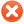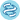Plot options# Use multiple genesUse list of genes Use mean expression of selected genes Use ratio of two genes Filter by median expression
more genes param

# ExampleExample input for multiple genes:
1007_s_at 1
200000_s_at -1
31637_s_at 0.5
40016_g_at -2

Explanation:
first: normal,
second: gene is inverted,
third: gene has weight,
fourth: gene has weight and is inverted

You can also use following format:
1007_s_at, 1
200000_s_at, -1
31637_s_at, 0.5
40016_g_at, -2

 Split patients by --none--lower quartilelower tertilemedianupper tertileupper quartile Auto select best cutoffTrichotomization : --none--T1 vs T3Q1 vs Q4Survival: RFS (n=1342)OS (n=551)PPS (n=145)Compute median survival:Follow up threshold: all2 months4 months6 months12 months18 months24 months30 months36 months48 months60 months120 months180 months240 monthsCensore at threshold:## Probe set options

Use
 user selected probe set all probe sets per geneonly JetSet best probe setPlot beeswarm graph of probe distribution:## Restrict analysis to subtypes...

 Stage: all each separately 1 (n=138) 2 (n=629) 1+2 (n=767) 3 (n=517) 1+2+3 (n=1284) 4 (n=216) 2+3+4 (n=1362) 3+4 (n=733) Location: all each separately proximal (n=481) distal (n=719) Site: all rectum (n=105) left colon (n=277) right colon (n=251) Stage T: all 2 (n=58) 3 (n=228) 4 (n=22) Stage N: all 0 (n=163) 1 (n=86) 2 (n=62) Stage M: all 0 (n=298) 1 (n=102) Grade: all each separately 1 (n=27) 2 (n=253) 3 (n=83) MSI: all stable (n=531) stable or low (n=602) high (n=247) BRAF mutation: all mutated (n=53) wild type (n=550) KRAS mutation: all mutated (n=252) wild type (n=384) TP53 mutation: all mutated (n=243) wild type (n=199)

## Restrict analysis to selected cohorts...

 Gender: all each separately female (n=959) male (n=858) CMS: all each separately microsatellite unstable (n=314) canonical (n=542) metabolic (n=370) mesenchymal (n=509) Adjuvant chemotherapy: all 0 (n=424) 1 (n=294)

## Use following dataset(s) for the analysis...

 Dataset: all each separately GSE12945 (n=62) GSE13294 (n=155) GSE14333 (n=123) GSE143985 (n=91) GSE17538 (n=232) GSE18088 (n=53) GSE26682 (n=331) GSE26906 (n=58) GSE30540 (n=35) GSE31595 (n=37) GSE33114 (n=90) GSE34489 (n=33) GSE37892 (n=130) GSE38832 (n=70) GSE39582 (n=514) GSE41258 (n=185)

Please note: the generated p value does not include correction for multiple hypothesis testing by default.Please kindly cite our paper to support further development: Lanczky A, Gyorffy B,. Web-Based Survival Analysis Tool Tailored for Medical Research (KMplot): Development and Implementation, J Med Internet Res, 2021 Jul 26;23(7):e27633.